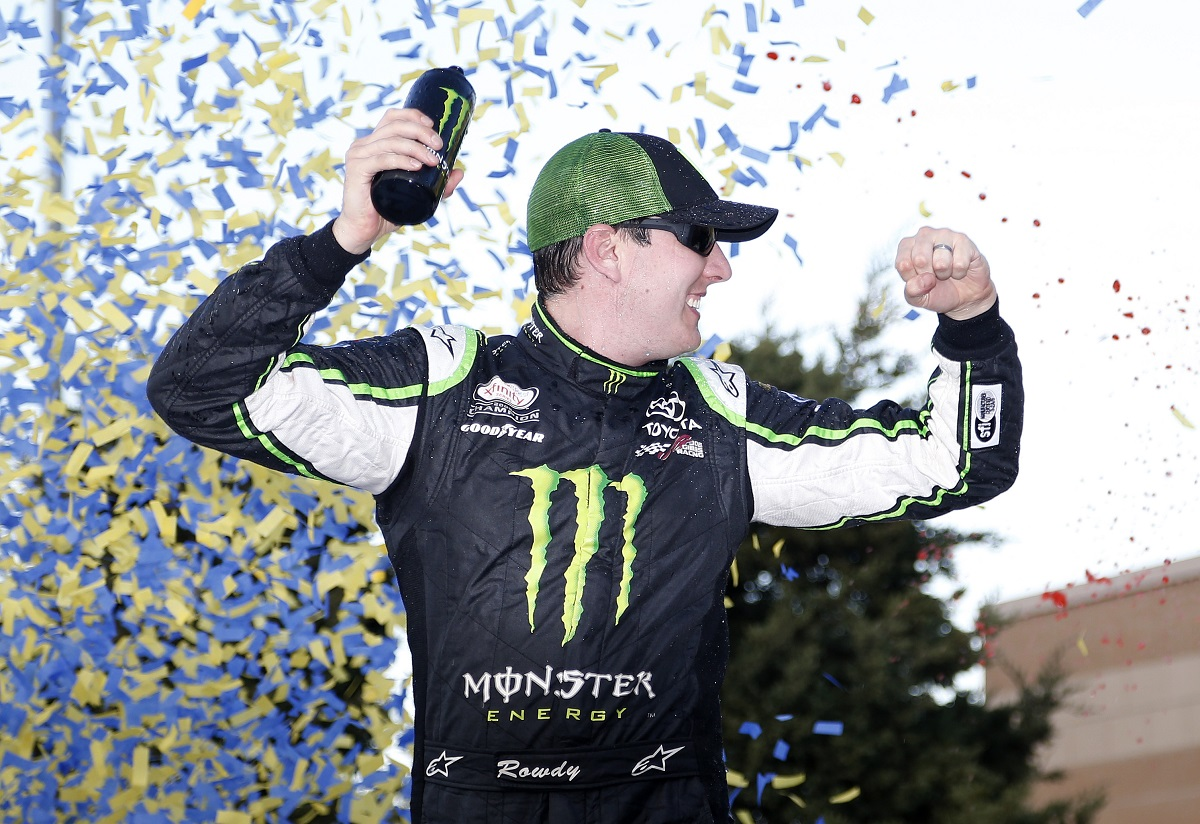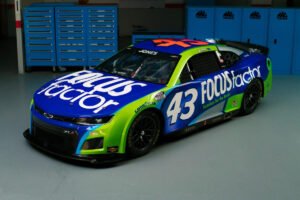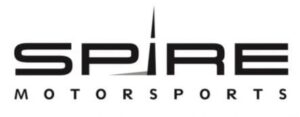November 29, 2021

# OnPitRoad.com Pick ‘Em Fantasy Pick Results for Kansas Lottery 300Photo Credit: Matt Sullivan/Getty Images

The Picks

Brandon Feeney, Ronald Costigan and Ashley McCubbin picked Chase Elliott, Kyle Busch and Kevin Harvick. Elliott finished seventh with Busch winning and Harvick 15th. Points: 37+47+29=113

Jeffrey Breazeale and Justin Tucker picked Regan Smith, Joey Logano and Kyle Busch. Smith finished fifth with Logano third. Points: 39+41+47=127

Robert Hill picked Darrell Wallace Jr., Matt Kenseth and Ryan Sieg. Wallace finished 11th with Kenseth second and Sieg eighth. Points: 33+44+36=115

Tim Johnston, Marshall Gabell and Brooke Clarke picked Chase Elliott, Joey Logano and Kevin Harvick, Points: 37+41+29=107

Kreistina Blinova picked Ty Dillon, Austin Dillon and Kevin Harvick. Ty finished fourth with Austin 16th. Points: 40+28+29=97

Kyle Magda picked Harrison Rhodes, Joey Logano and Daniel Suarez. Rhodes finished 27th with Suarez ninth. Points: 17+41+35=93

Steve Love picked Regan Smith, Matt Kenseth and Kevin Harvick. Points: 39+44+29=112

Jeni Harris picked Darrell Wallace Jr., Regan Smith and Chase Elliott. Points: 33+39+37=109

Doug Townsend picked Joey Logano, Kyle Busch and Chris Buescher. Buescher finished sixth. Points: 41+47+38=126

Parker Ledger picked Regan Smith, Chase Elliott and Kevin Harvick. Points: 39+37+29=105

Rory Townsend picked Chase Elliott, TJ Bell and Kevin Harvick. Bell finished 36th. Points: 37+8+29=74

Alex George picked Chase Elliott, Brennan Poole and Kevin Harvick. Poole finished 13th. Points: 37+31+29=97

Mike Cousins picked Ty Dillon, Chris Buescher and Kevin Harvick. Points: 40+38+29=107

Stephen Placido picked Darrell Wallace Jr., Joey Logano and Kevin Harvick. Points: 33+41+29=103

The Results

1. Jeffrey Breazeale and Justin Tucker – 127
2. Doug Townsend – 126
3. Robert Hill – 115
4. Brandon Feeney, Ronald Costigan and Ashley McCubbin – 113
5. Steve Love – 112
6. Jeni Harris – 109
7. Mike Cousins, Tim Johnston, Marshall Gabell and Brooke Clarke – 107
8. Parker Ledger – 105
9. Stephen Placido – 103
10. Alex George and Kreistina Blinova – 97
11. Kyle Magda – 93
12. Rory Townsend – 74

Current Fall XFINITY Series Standings

1. (Points Leader Post Chicago) Steve Love – 478+112=590
2. Justin Tucker – 451+127=578 (-12)
3. Jeffrey Breazeale – 443+127=570 (-20)
4. Brandon Feeney and Ronald Costigan – 450+113=563 (-27)
5. Jeni Harris – 451+109=560 (-30)
6. Doug Townsend – 429+126=555 (-35)
7. Ashley McCubbin – 430+113=543 (-47)
8. Robert Hill – 414+115=529 (-61)
9. Parker Ledger – 414+105=519 (-71)
10. Kreistina Blinova – 411+97=508 (-82)
11. Mike Cousins – 393+107=500 (-90)
12. Stephen Placido – 395+103=498 (-92)
13. Tim Johnston, Marshall Gabell and Brooke Clarke – 390+107=497 (-93)
14. Alex George – 368+97=465
15. Kyle Magda – 325+93=418
16. Rory Townend – 305+74=379

Full Season XFINITY Series Standings

2. Ronald Costigan – 3306+113=3419 (-55)
3. Jeffrey Breazeale – 3250+127=3377 (-97)
4. Ashley McCubbin – 3247+113=3360
5. Brandon Feeney – 3212+113=3325
6. Doug Townsend – 3173+126=3299
7. Steve Love – 3177+112=3289
8. Stephen Placido – 3069+103=3172
9. Brooke Clarke – 3062+107=3169
10. Tim Johnston – 3054+107=3161
11. Kyle Magda – 3032+93=3125
12. Jeni Harris – 3018+109=3127
13. Mike Cousins – 2969+107=3076
14. Parker Ledger – 2968+105=3073
15. Kreistina Blinova – 2944+97=3041
16. Marshall Gabell – 2932+107=3039
17. Robert Hill – 2894+115=3009
18. Alex George – 2772+97=2869
19. Rory Townsend – 2628+74=2702

Fall Overall Standings

1. (Points Leader Post Cup Charlotte) Steve Love – 1099+112=1211
2. Doug Townsend – 1065+126=1191 (-20)
3. Jeni Harris – 1072+109=1181 (-30)
4. Justin Tucker – 987+127=1114 (-97)
5. Brooke Clarke – 992+107=1099
6. Parker Ledger – 988+105=1093
7. Brandon Feeney – 976+113=1089
8. Ashley McCubbin – 971+113=1084
9. Ronald Costigan – 948+113=1061
10. Jeffrey Breazeale – 925+127=1052
11. Marshall Gabell – 940+107=1047
12. Stephen Placido – 937+103=1040
13. Mike Cousins – 931+107=1038
14. Tim Johnston – 930+107=1037
15. Kreistina Blinova – 937+97=1034
16. Rory Townsend – 913+74=987
17. Alex George – 869+97=966
18. Robert Hill – 849+115=964
19. Kyle Magda – 791+93=884

Full Season Overall Points Standings

1. (Points Leader Post Cup Kansas) Justin Tucker – 7753+127=7880
2. Ronald Costigan – 7579+113=7692 (-188)
3. Ashley McCubbin – 7545+113=7658
4. Doug Townsend – 7475+126=7601
5. Brandon Feeney – 7459+113=7572
6. Steve Love – 7435+112=7547
7. Stephen Placid0 – 7368+103=7471
8. Parker Ledger – 7275+105=7380
9. Brooke Clarke – 7353+107=7360
10. Tim Johnston – 7203+107=7310
11. Jeffrey Breazeale – 7177+127=7304
12. Mike Cousins – 7156+107=7263
13. Kyle Magda – 7115+93=7208
14. Kreistina Blinova – 7085+97=7182
15. Marshall Gabell – 7075+107=7182
16. Jeni Harris – 7025+109=7134
17. Alex George – 6969+97=7066
18. Rory Townend – 6760+74=6834
19. Robert Hill – 6371+115=6486

#### You may have missed#### Why Progressive Insurance is the right choice#### FOCUSFACTOR® TO PARTNER WITH RICHARD PETTY MOTORSPORTS#### Spire Motorsports to Field NASCAR Camping World Truck Series Team in 2022